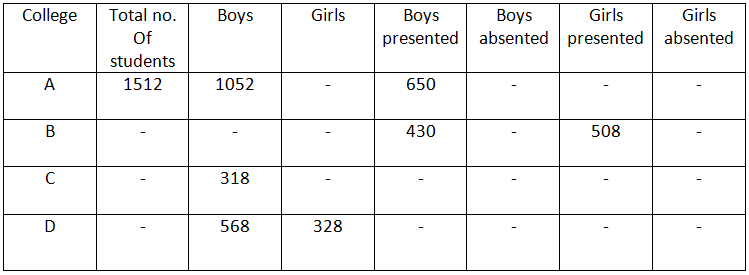## Questions On Quantitative Techniques SMQTQ012

Direction: The following table shows the Number of students who study in four different colleges in which some are boys and some are girls. Some values are missing in the table. Study the information given in the questions and find the missing values where needed and answer the questions based on it.Question 1.The number of boys who are absented in college B is 52 more than the number of boys who are presented in that college. The number of boys who are absented in college B is 244 more than the number of girls who are absented in that college. Find the total number of students in college B.

1. 1768
2. 1578
3. 1648
4. 1658

Question 2. The number of absented girls in college A is 4 more than half of the number of girls who are presented in the same college. Find the difference between number of boys who are absented in college A and number of girls who are also absented in that college.

1. 256
2. 246
3. 146
4. 250

Question 3. Number of girls in college C is 294 more than the number of girls in college A. If the sum of presented boys and presented girls in college C is 230. Find the sum of absented boys and absented girls in college C.

1. 842
2. 852
3. 832
4. 942

Question 4. If in college C 50% boys are present and in college D 165 boys are absent the find the average number of boys presented in all the college together.

1. 410
2. 410.5
3. 412.5
4. 415

Question 5. The Number of girls who are presented and who are absented in college D is equal. If the number of boys who are presented in college D is 20 more than number of girls who are presented in that college then what is difference between percentage of boys presented and girls absented in that college.

1. 25%
2. 21.6%
3. 19.4%
4. 17.6%

Solution:

1. Ans. 4.

Solution:
number of boys absented in college B= 430+52=482
Number of girls absented in college B= 482-244=238
Total number of students in college B= 430+482+508+238=1658
Option 4 is correct.

2. Ans. 2.

Solution:
let presented girls= 2x so absented girls= x+4
Total number of girls = 1512- 1052 =460
Therefore 2x+x+4=460
X= 152 so absented girls = 156
Absented boys =1052- 650= 402
Difference = 402-156=246
Option 2 is correct.

3. Ans. 1.

Solution:
Number of girls in college C = 1512- 1052+294 =754
Total number of students in college C = 318+ 754=1072
Total number of absented boys and girls= 1072- 230= 842
Option 1 is correct.

4. Ans. 2

Solution:
boy presented in college C=318/2= 159
In college D = 568-165= 403
Average= (650+430+159+403)/4= 410.5
Option 2 is correct.

5. Ans. 4.

Solution- number of girls presented/absented = 328/2= 164
Absent % of girls = 50%
Number of boys presented = 164+20= 184
Present % of boys = 184×100/568=32.39%
% difference = 50- 32.4= 17.6%
So option 4 is correct.